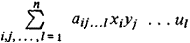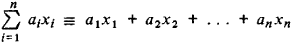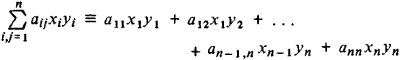# Multilinear Form

Also found in: Wikipedia.

## multilinear form

[′məl·tə‚lin·ē·ər ′fȯrm]
(mathematics)
A multilinear form of degree n is a polynomial expression which is linear in each of n variables.
McGraw-Hill Dictionary of Scientific & Technical Terms, 6E, Copyright © 2003 by The McGraw-Hill Companies, Inc.
The following article is from The Great Soviet Encyclopedia (1979). It might be outdated or ideologically biased.

## Multilinear Form

an algebraic expression of the formThis expression is a polynomial containing m sets of variables, with n variables in each set:

x1, x2, …, xn; y1, y2, …, yn; …; u1, u2, …, un

Each term of the polynomial is of the first degree in a variable from each set. A multilinear form is thus a linear polynomial expression in the variables of one set—hence its name. Special types of multilinear forms include the linear form (m = 1)the bilinear form (m = 2)and the trilinear form (m = 3).

The Great Soviet Encyclopedia, 3rd Edition (1970-1979). © 2010 The Gale Group, Inc. All rights reserved.
Mentioned in ?
References in periodicals archive ?
In representing the transnational networks of globalization, network films develop a multilinear form in which seemingly unrelated plots and protagonists are brought together by various means.
The most simple model of the relation between images and CGRs (usually taken as log(v)) may be expressed in a multilinear form (Lauschmann et al., 2002)
By using the alternation multilinear form, we can rewrite the elements from the wedge notation into tensor product by taking into account the signs of the permutations, so
Seeger, Smart, and Street introduce a class of multilinear singular integral forms that generalize the Christ-Journe multilinear forms. The research is partially motivated by an approach to Bressan's problems on incompressible mixing flows, they say, and a key aspect of the theory is that the class of operators is closed under adjoints, that is, the class of multilinear forms is closed under permutations of the entries.
Praciano-Pereira, On bounded multilinear forms on a class of [l.sup.p] spaces, J.
Maxwell's equations are derived by considering the canonical differential forms representing alternating multilinear forms which are obtained from the standard representation by the Hodge star operator.
Han, "Perron-Frobenius theorem for nonnegative multilinear forms and extensions," Linear Algebra and its Applications, vol.

Site: Follow: Share:
Open / Close